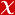## ON THE EXPONENTIAL DIOPHANTINE EQUATION (n-1)(x) + (n+2)(y) = n(z)

COLLOQUIUM MATHEMATICUM, vol.161, no.2, pp.239-249, 2020 (Journal Indexed in SCI)• Publication Type: Article / Article
• Volume: 161 Issue: 2
• Publication Date: 2020
• Doi Number: 10.4064/cm7668-6-2019
• Title of Journal : COLLOQUIUM MATHEMATICUM
• Page Numbers: pp.239-249
• Keywords: exponential Diophantine equation, primitive divisors of Lucas sequences, Jacobi symbol, lower bounds for linear forms in two logarithms, PRIMITIVE DIVISORS, LINEAR-FORMS, 2 LOGARITHMS, CONJECTURE, NUMBER, LUCAS

#### Abstract

Suppose that n is a positive integer. We show that the only positive integer solutions (n, x, y, z) of the exponential Diophantine equation